Courses

# Test: Heat Transfer By Forced Convection - 1

## 10 Questions MCQ Test Topicwise Question Bank for Mechanical Engineering | Test: Heat Transfer By Forced Convection - 1

Description
This mock test of Test: Heat Transfer By Forced Convection - 1 for Chemical Engineering helps you for every Chemical Engineering entrance exam. This contains 10 Multiple Choice Questions for Chemical Engineering Test: Heat Transfer By Forced Convection - 1 (mcq) to study with solutions a complete question bank. The solved questions answers in this Test: Heat Transfer By Forced Convection - 1 quiz give you a good mix of easy questions and tough questions. Chemical Engineering students definitely take this Test: Heat Transfer By Forced Convection - 1 exercise for a better result in the exam. You can find other Test: Heat Transfer By Forced Convection - 1 extra questions, long questions & short questions for Chemical Engineering on EduRev as well by searching above.
QUESTION: 1

Solution:
QUESTION: 2

### Which of the following dimensionless number/parameter is different from others?

Solution:

(b), (c) and (d) are dimensionless heat transfer coefficient

QUESTION: 3

### Match List-l with List-ll List-l A. Reynolds number B. Prandtl number C. Nusselt number D. Mach number List-lI 1. Film coefficient, pipe diameter, thermal conductivity 2. Flow velocity, acoustic velocity 3. Heat capacity, dynamic viscosity, thermal conductivity 4. Flow velocity, pipe diameter, kinematic viscosity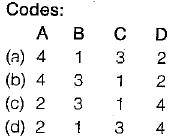Solution:
QUESTION: 4

Stanton number may be interpreted as the ratio of

Solution:
QUESTION: 5

The Nusselt number is related to Reynolds number in laminar and turbulent flows respectively as

Solution:

Nu = C Re0.5 Pr0.33 (for laminar flow) Nu = C Re0.8 Pr0.33 (for turbulent flow)

QUESTION: 6

For turbulent flow over a flat plate, the average value of Nusselt number is prescribed by the relation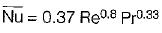Which of the followings is then a false statement? The average heat transfer coefficient increase as

Solution: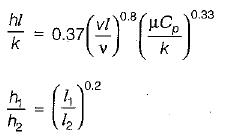∴ h2 decreases as 1/5 power of plate length.

QUESTION: 7

Reynolds analogy states that

Solution:
QUESTION: 8

The flow and heat transfer in coiled tubes are governed by

Solution:

The flow and heat transfer in coiled tubes are governed by Dean number,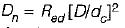Where D is tube diameter
dc is coil diameter

QUESTION: 9

Which one of the following is flow properly

Solution:

Reynolds number is flow property depends on velocity of flow. It is not the property of fluid.

QUESTION: 10

For a fluid having Prandtl number equal to unity how are the hydrodynamic boundary layer thickness δ and the thermal boundary layer thickness δt related

Solution: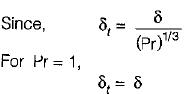Where,
δt = Thickness of Thermal boundary layer
δ = Thickness of hydrodynamic boundary layer